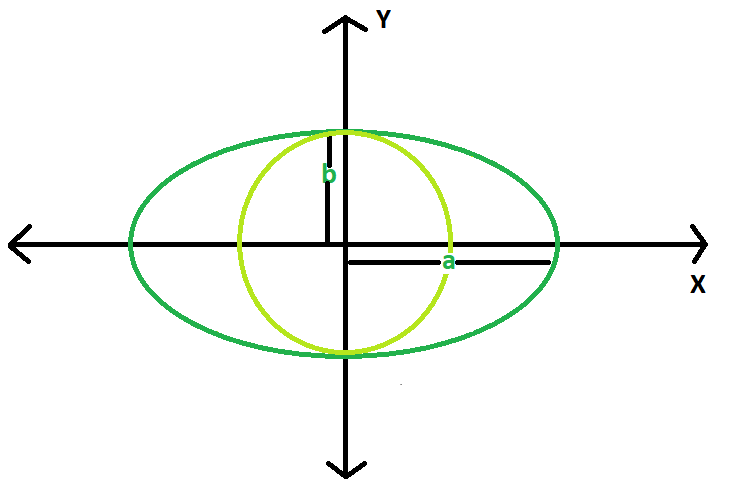# Find the area of largest circle inscribed in ellipse

• Last Updated : 18 Mar, 2021

Given an ellipse, with major and minor axis length 2a & 2b respectively. The task is to find the area of the largest circle that can be inscribed in it.
Examples:

```Input : a = 5, b = 3
Output : 28.2743

Input : a = 10, b = 8
Output : 201.062```

Attention reader! Don’t stop learning now. Get hold of all the important DSA concepts with the DSA Self Paced Course at a student-friendly price and become industry ready.  To complete your preparation from learning a language to DS Algo and many more,  please refer Complete Interview Preparation Course.

In case you wish to attend live classes with experts, please refer DSA Live Classes for Working Professionals and Competitive Programming Live for Students.Approach : The maximal radius of the circle inscribed in the ellipse is the minor axis of the ellipse.
So, area of the largest circle = π * b * b.
Below is the implementation of the above approach:

## C++

 `// CPP program to find``// the area of the circle``#include ``using` `namespace` `std;``#define pi 3.1415926` `double` `areaCircle(``double` `b)``{``    ``double` `area = pi * b * b;``    ``return` `area;``}` `// Driver Code``int` `main()``{``    ``double` `a = 10, b = 8;``    ``cout << areaCircle(b);``    ``return` `0;``}`

## Java

 `// Java Program to find the area``// of circle` `class` `GFG``{``    ``static` `double` `areaCircle(``double` `b)``    ``{``    ` `    ` `        ``// Area of the Reuleaux triangle``        ``double` `area = (``double``)``3.1415926` `* b * b;``        ``return` `area;``    ``}``    ` `    ``// Driver code``    ``public` `static` `void` `main(String args[])``    ``{``        ``float` `a = ``10``,b = ``8``;``        ``System.out.println(areaCircle(b)) ;``    ``}``}` `// This code is contributed by mohit kumar 29`

## Python3

 `# Python3 program implementation of above approach` `import` `math` `# Function to return required answer``def` `areaCircle(b):``    ``area ``=` `math.pi ``*` `b ``*` `b``    ``return` `area`  `# Driver Code``a ``=` `10``b ``=` `8``print``(areaCircle(b))` `# This code is contributed by``# Sanjit_Prasad`

## C#

 `// C# Program to find the area``// of circle``using` `System;` `class` `GFG``{``    ``static` `double` `areaCircle(``double` `b)``    ``{``        ``// Area of the Reuleaux triangle``        ``double` `area = (``double``)3.1415926 * b * b;``        ``return` `area;``    ``}``    ` `    ``// Driver code``    ``public` `static` `void` `Main()``    ``{``        ``float` `b = 8;``        ``Console.WriteLine(areaCircle(b)) ;``    ``}``}` `// This code is contributed by aishwarya.27`

## PHP

 ``

## Javascript

 ``
Output:
`201.062`

My Personal Notes arrow_drop_up